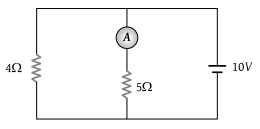# NEET Questions Solved

In the circuit, the reading of the ammeter is (assume internal resistance of the battery be zero)(1) $\frac{40}{29}A$

(2) $\frac{10}{9}A$

(3) $\frac{5}{3}A$

(4) 2 A

(4) Applying Kirchhoff law in the first mesh

$10=5×i$$i=\frac{10}{5}=2\text{\hspace{0.17em}}A$

Difficulty Level:

• 7%
• 23%
• 10%
• 62%